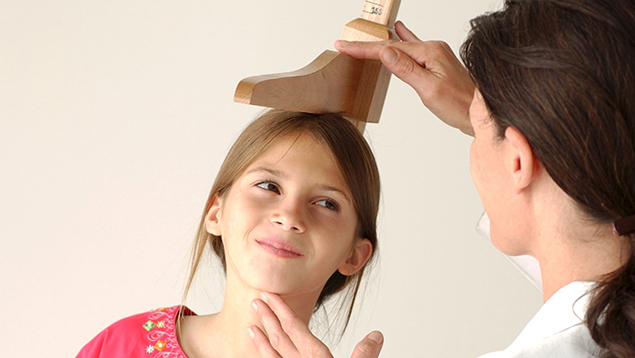# Use This Simple Trick To Work Out How Tall Your Kids Will Be

Publish Date
Friday, 6 May 2016, 2:36PMCurious about how tall your kids are going to be? Well apparently there is a mathematical equation to figure it out...

For boys, the formula is = combine the height of both parents, add 13 centimeters (5 inches) and divide by two.

For girls, the formula is = combines the height of the parents, subtracts 13 centimeters (five inches) and divide by two.

Note that there is a more complex formula accounts for extremes in parental height.

It worked for me, but let us know if you try it and how it goes.